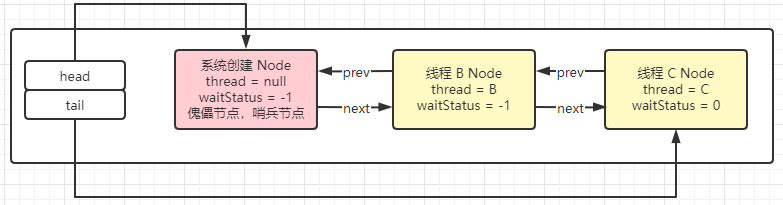# AQS 源码解读之加锁篇## 分析 demo

``````public class AQSDemo {
// 带入一个银行办理业务的案例
public static void main(String[] args) {
// 创建一个非公平锁
ReentrantLock lock = new ReentrantLock();
// 三个线程模拟3个网点
// A 顾客就是第一个顾客，此时没有没有其他顾客
lock.lock();
try {
try {
TimeUnit.MINUTES.sleep(20);
} catch (InterruptedException e) {
e.printStackTrace();
}
} catch (Exception e) {
e.printStackTrace();
} finally {
lock.unlock();
}
}, "A").start();

// 第二个线程 --> 由于受理窗口只有一个（只能一个线程持有锁），此时 B 只能等待
// 进入候客区
lock.lock();
try {
} catch (Exception e) {
e.printStackTrace();
} finally {
lock.unlock();
}
}, "B").start();

// 第三个线程 --> 由于受理窗口只有一个（只能一个线程持有锁），此时 B 只能等待
// 进入候客区
lock.lock();
try {
} catch (Exception e) {
e.printStackTrace();
} finally {
lock.unlock();
}
}, "C").start();
}
}
``````

## 线程 A

### lock 方法分析

#### 第一步：

``````public void lock() {
sync.lock();
}
``````

#### 第二步：

##### 抽象类 sync 的具体实现
``````static final class NonfairSync extends Sync {
private static final long serialVersionUID = 7316153563782823691L;

final void lock() {
if (compareAndSetState(0, 1))
else
acquire(1);
}

protected final boolean tryAcquire(int acquires) {
return nonfairTryAcquire(acquires);
}
}
``````
##### 执行 compareAndSetState(0, 1) 方法
``````protected final boolean compareAndSetState(int expect, int update) {
return unsafe.compareAndSwapInt(this, stateOffset, expect, update);
}
``````

``````protected final void setExclusiveOwnerThread(Thread thread) {
}
``````

## 线程 B

### lock 方法分析

#### 第二步：

##### 2.1 抽象类 sync 的具体实现
``````final void lock() {
if (compareAndSetState(0, 1))
else
acquire(1);
}
``````
##### 2.2 执行 compareAndSetState(0, 1) 方法
``````// expect = 0 update = 1
protected final boolean compareAndSetState(int expect, int update) {
return unsafe.compareAndSwapInt(this, stateOffset, expect, update);
}
``````

##### 2.3 执行 acquire(1) 方法 1197
``````// arg = 1
public final void acquire(int arg) {
if (!tryAcquire(arg) &&
selfInterrupt();
}
``````
##### 2.4 执行 !tryAcquire(arg) 方法
###### 2.4.1 此方法是 AQS 中的抽象类，需要查看器具体实现

``````protected boolean tryAcquire(int arg) {
throw new UnsupportedOperationException();
}
``````
###### 2.4.2 找到具体实现，在 NonfairSync 类中，上面的代码可以 [点击查看](##### 2.1 抽象类 sync 的具体实现)。
``````// acquires = 1
protected final boolean tryAcquire(int acquires) {
return nonfairTryAcquire(acquires);
}
``````
##### 2.5 执行了 nonfairTryAcquire(acquires) 方法
``````// acquires = 1
final boolean nonfairTryAcquire(int acquires) {
// getState() 方法返回 1，因为 state 已经被第一个线程所修改了
int c = getState();
if (c == 0) {
if (compareAndSetState(0, acquires)) {
return true;
}
}
// 此时如果线程一再次过来获取到锁，就可以直接进去也就是可重入锁
else if (current == getExclusiveOwnerThread()) {
int nextc = c + acquires;
if (nextc < 0) // overflow
throw new Error("Maximum lock count exceeded");
setState(nextc);
return true;
}
return false;
}
``````

nonfairTryAcquire 方法首先校验了 state 的值是否等于 0，也就是看看上一个占用锁的线程是不是已经把资源给释放了。

Node.EXCLUSIVE：static final Node EXCLUSIVE = null; 也就是排他的意思。

``````// mode = null
// 创建一个 Node 节点
// tail：尾节点，此时为 null
Node pred = tail;
if (pred != null) {
node.prev = pred;
if (compareAndSetTail(pred, node)) {
pred.next = node;
return node;
}
}
enq(node);
return node;
}
``````
``````// thread = 线程二 mode = null
// 将当前等待节点谁知为 null
this.nextWaiter = mode;
// 这个 Node 节点的线程设置为 线程二
}
``````
###### 2.6.2 enq(node)
``````// node 等于 2.6.1 创建的 Node 节点
private Node enq(final Node node) {
// 自旋操作
for (;;) {
// 此时尾结点 tail 为 null
Node t = tail;
// t is null 进行初始化操作，这一步也就是意味着此时 CLH 队列中还没有任何一个元素。
if (t == null) {
// 成功将队列里面的头节点替换成新创建的 Node 节点
// 将尾结点也指向新创建 Node 节点
} else {
node.prev = t;
if (compareAndSetTail(t, node)) {
t.next = node;
return t;
}
}
}
}
``````
``````private final boolean compareAndSetHead(Node update) {
}
``````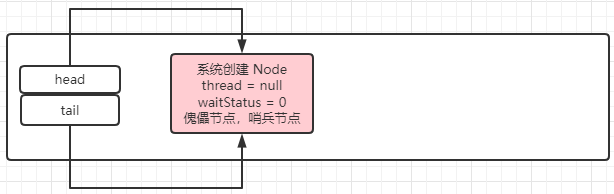###### 2.6.4 enq(node) 第二次循环
``````// node = 2.6.1 创建的 Node 节点，也就是线程二的 Node 节点
private Node enq(final Node node) {
// 自旋操作
for (;;) {
// 此时尾结点 tail 为 新创建的 node 节点
Node t = tail;
// t is not null 执行 else
if (t == null) {
} else {
// 将 node 节点的前指针指向新创建的头节点
node.prev = t;
// 通过比较替换将队列的尾结点替换了线程 B 的 Node 节点
if (compareAndSetTail(t, node)) {
// 将系统初始的 Node 的后指针指向线程 B 的 Node 节点
t.next = node;
// 然后返回 B 线程的 Node 节点
return t;
}
}
}
}
``````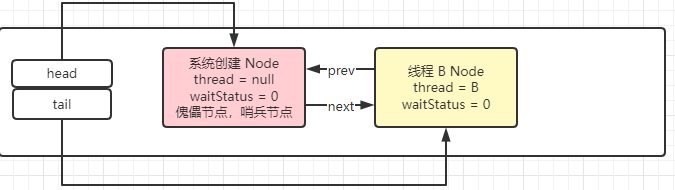``````// node：线程 B 对应的 Node 节点  arg = 1
final boolean acquireQueued(final Node node, int arg) {
boolean failed = true;
try {
boolean interrupted = false;
// 自旋操作
for (;;) {
// 获取现在队列中的第一个节点也就是系统创建的 Node 节点
final Node p = node.predecessor();
// P 现在是头节点，true。但是线程 B 尝试获取锁失败，false
if (p == head && tryAcquire(arg)) {
p.next = null; // help GC
failed = false;
return interrupted;
}
// 所以执行这一步，返回 false。所以进行下一次循环
if (shouldParkAfterFailedAcquire(p, node) &&
parkAndCheckInterrupt())
interrupted = true;
}
} finally {
if (failed)
cancelAcquire(node);
}
}
``````
###### 2.7.1 node.predecessor();
``````final Node predecessor() throws NullPointerException {
Node p = prev;
if (p == null)
throw new NullPointerException();
else
return p;
}
``````

###### 2.7.3 shouldParkAfterFailedAcquire(p, node)

``````// pred 系统创建的 Node 节点，node 线程 B 对应的 Node 节点
private static boolean shouldParkAfterFailedAcquire(Node pred, Node node) {
// 此时头节点的 waitStatus = 0
int ws = pred.waitStatus;
// Node.SIGNAL = -1
if (ws == Node.SIGNAL)
return true;
if (ws > 0) {
do {
node.prev = pred = pred.prev;
} while (pred.waitStatus > 0);
pred.next = node;
} else {
// 通过比较替换，将头节点的值从 0 调整为 -1
compareAndSetWaitStatus(pred, ws, Node.SIGNAL);
}
return false;
}
``````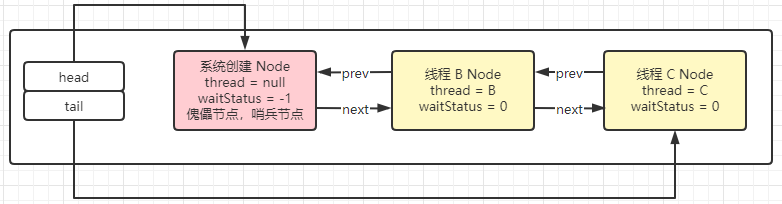###### 2.7.4 shouldParkAfterFailedAcquire(p, node)

``````// node：线程 B 对应的 Node 节点  arg = 1
final boolean acquireQueued(final Node node, int arg) {
boolean failed = true;
try {
boolean interrupted = false;
// 自旋操作
for (;;) {
// 获取现在队列中的第一个节点也就是系统创建的 Node 节点
final Node p = node.predecessor();
// P 现在是头节点，true。但是线程 B 尝试获取锁失败，false
if (p == head && tryAcquire(arg)) {
p.next = null; // help GC
failed = false;
return interrupted;
}
// 所以第二次循环后返回 ture，执行下一步
if (shouldParkAfterFailedAcquire(p, node) &&
parkAndCheckInterrupt())
interrupted = true;
}
} finally {
if (failed)
cancelAcquire(node);
}
}
``````
``````// pred 系统创建的 Node 节点，node 线程 B 对应的 Node 节点
private static boolean shouldParkAfterFailedAcquire(Node pred, Node node) {
// 经过第一次循环操作，此时头节点的 waitStatus = -1
int ws = pred.waitStatus;
// Node.SIGNAL = -1
if (ws == Node.SIGNAL)
return true;
if (ws > 0) {
do {
node.prev = pred = pred.prev;
} while (pred.waitStatus > 0);
pred.next = node;
} else {
// 通过比较替换，将头节点的值从 0 调整为 -1
compareAndSetWaitStatus(pred, ws, Node.SIGNAL);
}
return false;
}
``````
###### 2.7.5 parkAndCheckInterrupt()
``````// this 当前 B 线程
private final boolean parkAndCheckInterrupt() {
// 将当前线程 B 进行挂起
LockSupport.park(this);
}
``````

## 线程 C

### lock 方法分析

``````// mode 还是等于 null 排他
// 创建线程 C 的 Node 节点
// 此时尾指针指向的是线程 B 的 Node 节点
Node pred = tail;
if (pred != null) {
// 将线程 C 的 Node 节点的前指针指向线程 B 的 Node 节点
node.prev = pred;
// 通过比较替换将线程的尾指针指向线程 C 的 Node 节点
if (compareAndSetTail(pred, node)) {
// 将线程 B 的 Node 节点的后指针指向线程 C 的 Node 节点
pred.next = node;
// 返回线程 C 的 Node 节点
return node;
}
}
enq(node);
return node;
}
``````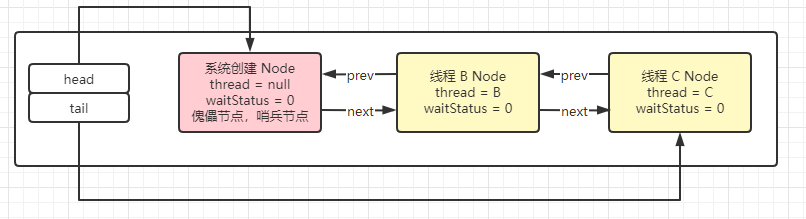``````// node 线程 c 对应的 Node  arg = 1
final boolean acquireQueued(final Node node, int arg) {
boolean failed = true;
try {
boolean interrupted = false;
for (;;) {
// 获取线程 c 对应的 Node 节点的前指针对应的 Node(线程 B 的 Node 节点线程 B 的 Node 节点)
final Node p = node.predecessor();
if (p == head && tryAcquire(arg)) {
p.next = null; // help GC
failed = false;
return interrupted;
}
//
if (shouldParkAfterFailedAcquire(p, node) &&
parkAndCheckInterrupt())
interrupted = true;
}
} finally {
if (failed)
cancelAcquire(node);
}
}
``````

#### shouldParkAfterFailedAcquire(p, node)

##### 第一次执行
``````// pred 线程 B 对应的 Node    node 线程 c 对应的 Node
private static boolean shouldParkAfterFailedAcquire(Node pred, Node node) {
// 此时线程 B 的 waitStatus = 0
int ws = pred.waitStatus;
if (ws == Node.SIGNAL)
return true;
if (ws > 0) {
do {
node.prev = pred = pred.prev;
} while (pred.waitStatus > 0);
pred.next = node;
} else {
// 通过比较替换将线程 B 的 waitStatus 改成 -1
compareAndSetWaitStatus(pred, ws, Node.SIGNAL);
}
return false;
}
``````
##### 第二次执行
``````// pred 线程 B 对应的 Node    node 线程 c 对应的 Node
private static boolean shouldParkAfterFailedAcquire(Node pred, Node node) {
// 此时线程 B 的 waitStatus = -1
int ws = pred.waitStatus;
if (ws == Node.SIGNAL)
return true;
if (ws > 0) {
do {
node.prev = pred = pred.prev;
} while (pred.waitStatus > 0);
pred.next = node;
} else {
compareAndSetWaitStatus(pred, ws, Node.SIGNAL);
}
return false;
}
``````

#### parkAndCheckInterrupt()

``````private final boolean parkAndCheckInterrupt() {
LockSupport.park(this);
}
``````